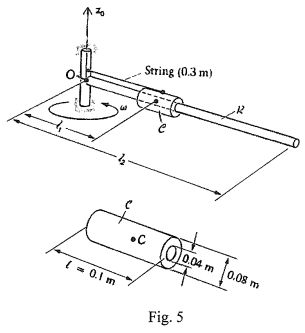milk>試卷(2021/09/17)

# 110 年 - 110 國立臺灣大學_碩士班招生考試_機械工程研究所機械製造組:應用力學(C)(包含靜力學、動力學）#101324

【非選題】
1.

1. (20%) The compound beam shown in Fig. I is pin-connected at B. Determine the reaction at the support A. Please neglect its weight and thickness.【非選題】
2.

2. (20%) At the instant shown in Fig. 2, the velocity of point A is 0.2 m/s to the right. Find the angular velocity of rod B, and determine the velocity of its other end (point B) which is constraint to move in the circular slot.【非選題】
3.

3. (20%) Collar Cin Fig. 3 is pined to rod Rat P and is free to slide along rod B. The angular velocity of Ris 0.2 rad/s in clockwise direction. Find the angular velocity of B at this time. Determine the velocity of P relative to B.【非選題】
4.

4. (20%) As shown in Fig. 4, force P is applied to a plate that rests on a smooth surface with the friction coefficient μ2equal to 0. The friction coefficient between the Mass m and Mass M is given as μ1. Find the largest force P for which the pipe will not slip on the plate.【非選題】
5.

5. (20%) The 2-kg collar C in Fig. 5 turns along the smooth rod R. which is  l2=I m long, has a mass of 3 kg, and is mounted in bearing with negligible friction. The angular speed is increased until the string located at lI=0.3m breaks (its tensile strength is 60N) and at that instant the external moment is removed.【題組】 (a) (10%) Determine the angular velocity of C when the string breaks.

【非選題】
6.【題組】(b) (10%) Determine the angular velocity of Rand the velocity of C (the mass center of C) when the collar leaves the rod.

### 懸賞詳解

#### 國二國文上第三次

13.請依序填入相似字形：□有所成、□心耿耿、情有獨□、無動於□。 (A)終、忠、鍾、衷　　 　 (B)終、忠、鐘、盅 (C)中、衷、鐘、盅　　...